CHARTWORK

### Explain with the use of a diagram the Earth's Magnetic Lines.### Explain Magnetic Variation.

Magnetic Variation ; Angle East or West between True North and Magnetic North### Explain with the use of a compass rose Variation.

Variation### Explain with the use of a diagram the approximate area where a Magnetic Compass is unstable.

Unstable area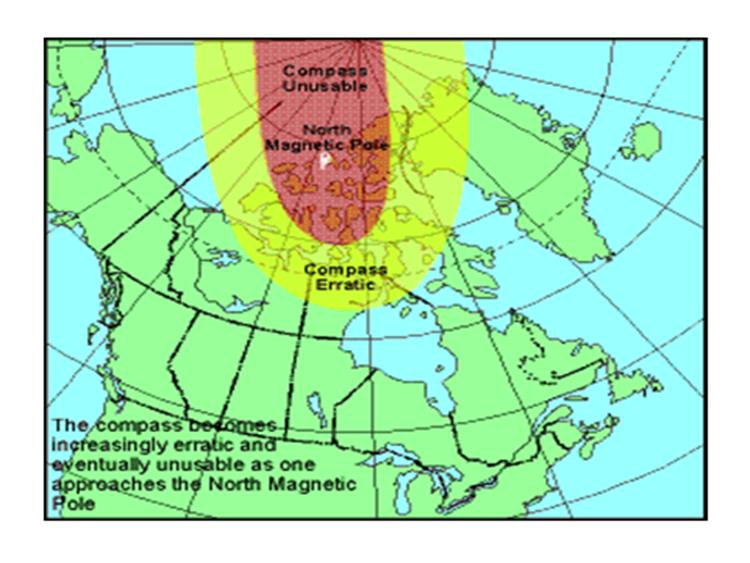### Explain why a Boat Compass does not point exactly towards Magnetic North.

The Boat Compass does not point exactly towards the Magnetic North, because it is affected by ferrous masses and electric currents in the vacinity of the compass.

Electric wires, portable radios, binoculars, tools and other fero-magnetic objects will affect the boat compass.### Explain Compass Deviation.

Compass Deviation; Angle East or West between Magnetic North and Boat Compass.

### Explain with use of a diagram Compass Deviation.

Compass Deviation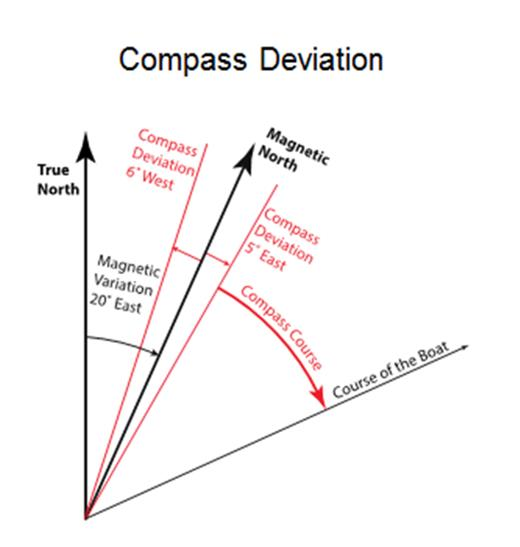### What causes Compass Deviation?

Causes of Compass Deviation

Local magnetic fields:

• Magnetic masses (winches / magnets from loud speakers / nearby radios / tools, etc.)
• Electric currents producing a magnetic filed (lights / remote controls, etc.)

### Explain Conversions between the Norths.

Conversions between the Norths

• True to Magnetic / Magnetic to True
• Magnetic to Compass / Compass to Magnetic
• True to Compass / Compass to True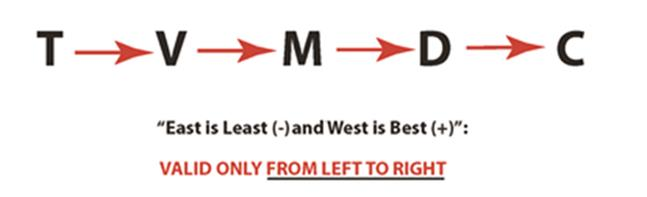### When taking 2 bearings what results give the best accuracy?

2 LOP at right angles to each other. To obtain more accurate results 3 LOP should be taken if possible to eliminate any errors that may occur from taking 2 LOP.

2 bearing LOP errors;

- Error in identifying the landmark (wrong lighthouse)

- Error in the concersion from Magnetic to True### When taking LOP what consideration should be taken to obtain the most accurate results.

For best results 3 LOP should be taken at about 120 degrees. Taking a 3 bearing LOP eliminates errors than can occur with a 2 bearing LOP.

2 bearing LOP errors;

- Error in identifying the landmark (wrong lighthouse)

- Error in the concersion from Magnetic to True### Explain how you would determine your distance from a landmark by doubling your bearing.

Determining the distance from a landmark

Using a hand bearing compass, a pelorus, or an Automatic Direction Finder (ADF)

Doubling the bearing, e.g. from 30° to 60°:

Distance from the landmark (BC) = distance travelled by the boat (AB)### Explain how you would determine your distance from a landmark by doubling your bearing.

Doubling the bearing. ie. from 45° to 90°:

Distance from the landmark (BC) = distance travelled by the boat (AB)### Determine the distance off a landmark using distance travelled.

Distance from the landmark ≈ 6 times the distance travelled (A-B) for a change of bearing of 10°.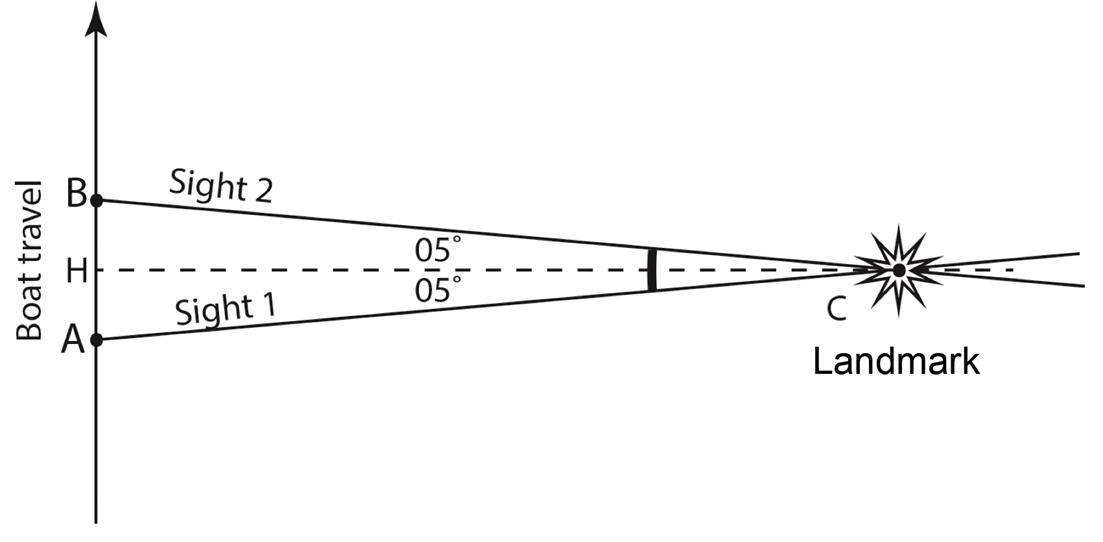### Explain distance off by Vertical Sextant Angle.

Vertical Sextant angle.

d = h / tg α Measure α with a vertical sextant;

- read “tg α” off a trigonometric table

- read h off the marine chart

- calculation = d### Explain how to calculate the position of your vessel by using a vertical sextant angle and a bearing.

The boat position can be determined from a distance to the landmark (circle of position, vertical sextant), and a bearing (hand bearing compass)### Explain how to determine your position using 3 landmarks with horizontal sextant angles.

The boat position can be established from two circles of position (horizontal sextant angles, using three landmarks.### Explain the GPS accuracy on electronic charts.

Under the best conditions the horizontal accuracy of the GPS system is approximately 3 to 8 metres 95% of the time

The use of the Wide Area Augmentation System (WAAS), which provides corrections through a geostationary satellite, is one way to increase accuracy. Differential GPS, sending local corrections via ground transmitters close to US and Canadian shorelines, is another way.

Vertical accuracy is considerably lower.

### Explain the Limiting Factors of using GPS.

Limiting Factors of using GPS; - Ionospheric and tropospheric interference - Satellite positioning - Calculating ability and accuracy of the GPS unit - Multipath signals; Natural or artificial interference - Horizontal chart datum and chart reliability - Disappearance of details in vector charts at small scales - Loss of signal due to accidental disconnection - Loss of signal due to automatic cut-off at slow speed

### With the use of a diagram explain the cycle of tides.

Tide cycle.### Explain tides. Duration / Time Intervals / Range / Height.

Tides;

- Duration of Tide : time between Hi & Lo tides around measurement time.

- Time Intervals : time between measurement time and nearest Hi or Lo.

- Range of tide : height difference between Hi & Lo.

- Height Difference : height difference between height at measurement and nearest Hi or Lo.

### Explain how to determine the height of tides in feet without tide tables.

The rule of incremental 12ths;

– Particularly useful for tides in feet – Assumes: Tide duration = 6 hours, Change in heights before or after a High or a Low =

• 1/12th of the total tide range during the first hour

• 2/12th during the second hour

• 3/12th during the third hour, and then the reverse: 3, 2, and 1 twelfths.

Height at any time = sum of the 12ths of the range accumulated since the High or the Low tide used as a reference.

Example:

1/12, 2/12, 3/12

- Falling tide - Range = 11 feet (difference between the height of the High and the height of the following Low). - Drop, four hours after the High? - (1/12 + 2/12 + 3/12 + 3/12) x 11' = 9/12 x 11' = 99'/12 = 99 inches, or 8.25'

### Explain how to determine the height of tides in meters without tide tables.

The rule of 10ths and Quarters: 1/10, 1/4, 2/4, 3/4, 9/10

- Particularly useful for tides in meters - Assumes: Tide duration = 6 hours,
Change in heights before or after a High or a Low =

• 1/10th of the total tide range after one hour
• 1/4 after two hours
• 2/4 after three hours
• 3/4 after four hours
• 9/10th after five hours

- Gives directly the height of the tide, without adding fractions.

- More precise, close to a High or a Low, than the method of the 12ths.

Example:

1/10, 1/4, 2/4, 3/4, 9/10

- Rising tide - Range = 6 m - Tide, four hours after the Low? • 4 hours after the Low = 2 hours before the next High • Tide height 4 hours after the low = 3/4 x (6m) = 4.5 m

### Explain a Vector.

A vector is a way to represent a phenomenon which can be defined by;

- A direction - A magnitude (intensity)

Examples:

- A movement (direction, and distance travelled) - A speed (direction, and magnitude) - A force (direction, and magnitude)

### Explain how to determine Resulting Speed using vectors.

Resulting speed: Place the two speed vectors (“distance travelled” in one hour) at the end of each other.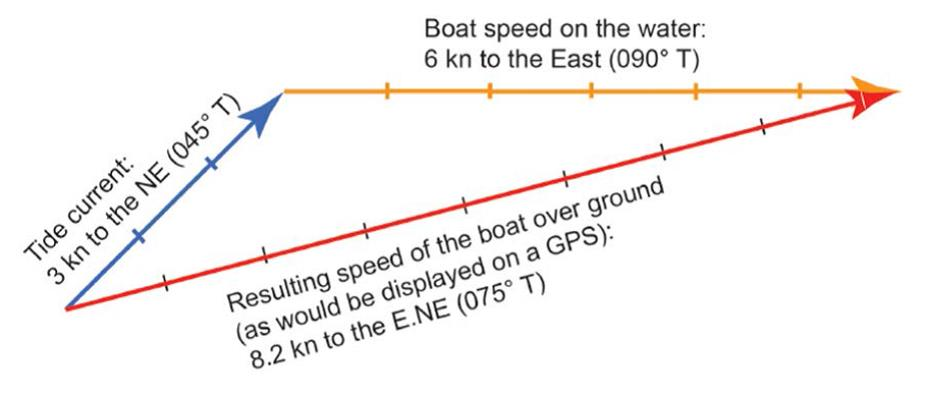### Explain how to determine Resulting Distance Travelled using vectors.

Resulting distance travelled: Place the two vectors representing the “distance travelled” during the time considered (e.g. 50 min or 1 h 40 min) at the end of each other.### Explain with the use of a diagram Set and Drift.

Measuring current Direction (“Set”) and Speed (“Drift”)​​​​​​​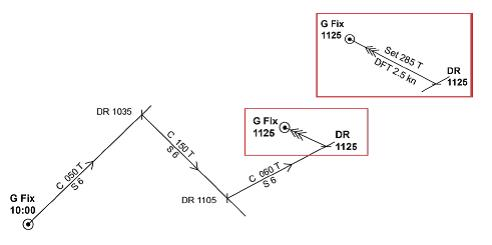### With the use of a diagram explain how to compensate for a Known Current.

Compensating for known current;

The vector construction is for one hour. Given the boat speed of 6 kn (arc of circle, 6 NM radius), the boat direction is chosen to offset the effect of the current (AC) and bring the boat back to the desired track (AB).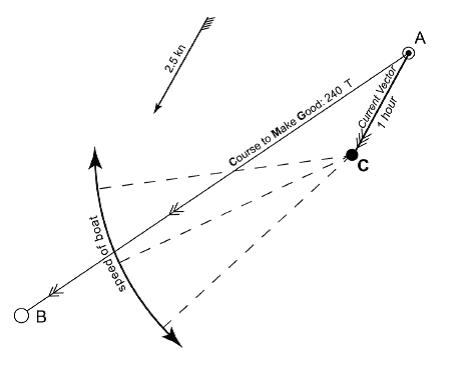### Explain with the use of a diagram how to compensate for leeway.

Leeway to port (wind on the starboard side)### Explain Nautical Miles

Distances are measured in Nautical Miles

- Abbreviation: “M” or, preferably, “NM”. - Original Definition: 1’ (from the Center of the earth) at the surface of the earth. - Only the Parallels of Latitude have a constant separation. Therefore, 1 NM = 1’ of Latitude - Current definition: 1,852 m

PDF file of

Chart symbols & abbreviations

- click to view -